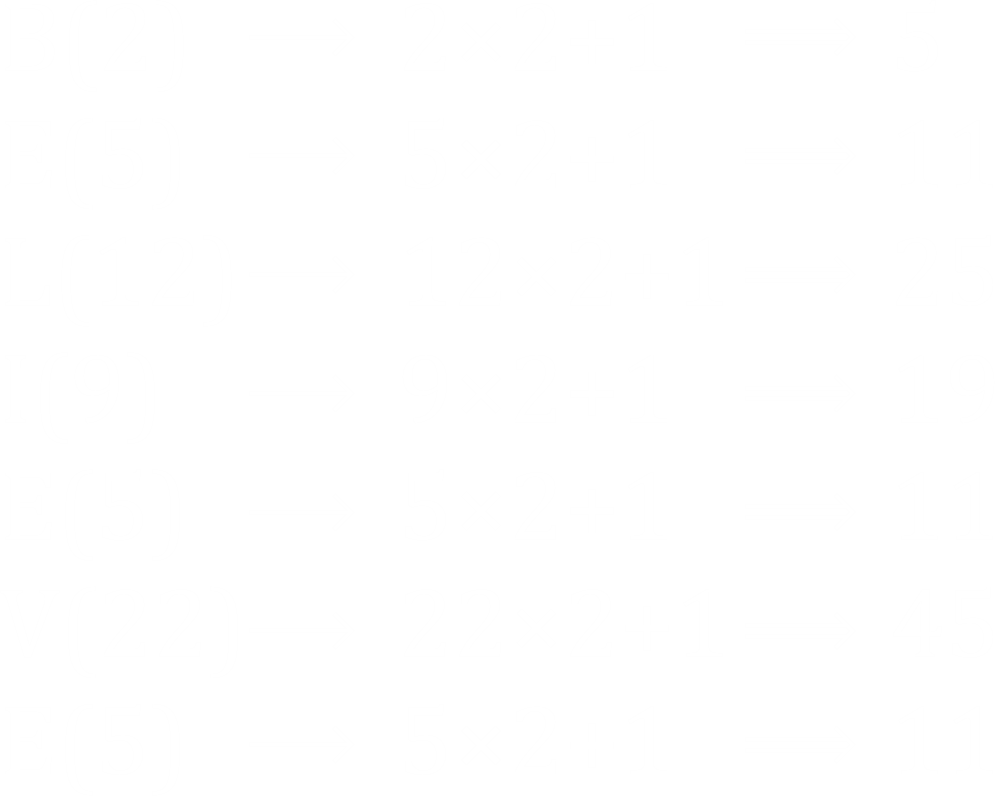Question of The Day12-03-2020

If code for A = 3, B = 5, and C = 7; then what will be the code for ‘BELIEVE’?

Correct Answer : a ) 5 -11-25-19-11-45-11

Explanation :

Let us assume A is numbered as 1, B as 2 and so on till Z being numbered as 26

The common logic for the codes of A, B and C is that the code for any alphabet can be given as the sum of twice the place value of that alphabet and one

As, A = 1 * 2 + 1 = 3

B = 2 * 2 + 1 = 5

C = 3 * 2 + 1 = 7

Therefore, BELIEVE will be coded asCode for ‘BELIEVE’ ⇒ 5 -11-25-19-11-45-11

Hence, (a) is the correct answer.

Such type of question is asked in various government exams like SSC CGL, SSC MTS, SSC CPO, SSC CHSL, RRB JE, RRB NTPC, RRB GROUP D, RRB OFFICER SCALE-I, etc.

Read Daily Current Affairs, Banking Awareness, Hindi Current Affairs, Word of the Day, and attempt free mock tests at PendulumEdu and boost your preparation for the actual exam.0